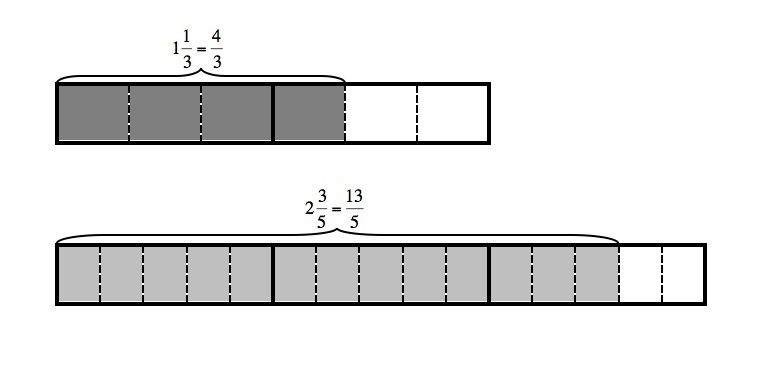# Mixed Numbers with Unlike Denominators

Alignments to Content Standards: 5.NF.A.1

Find two different ways to add these two numbers:

$$1\frac{1}{3} + 2\frac{3}{5}$$

## IM Commentary

The purpose of this task is to help students realize there are different ways to add mixed numbers and is most appropriate for use in an instructional setting. The two primary ways one can expect students to add are converting the mixed numbers to fractions greater than 1 or adding the whole numbers and fractional parts separately. It is good for students to develop a sense of which approach would be better in a particular context.

Note that the solutions show the sum as a single fraction and as a mixed number. While both are perfectly acceptable ways to write the sum, students should be encouraged to check to see that the two methods yield the same answer. Some students will convert the fraction to a mixed number, and some will write the mixed number as an equivalent fraction. Teachers should encourage both approaches.

## Solution

1. One way to add these numbers is to first convert the mixed numbers into equivalent fractions:

\begin{align} 1\frac{1}{3}&=\frac{1}{1}+\frac{1}{3}\\ &=\frac{1\times3}{1\times3}+\frac{1}{3}\\ &=\frac{3+1}{3}\\ &=\frac{4}{3} \end{align}

and

\begin{align} 2\frac{3}{5} &= \frac{2}{1}+\frac{3}{5}\\ &= \frac{2\times5}{1\times5}+\frac{3}{5} \\ &= \frac{10+3}{5} \\ &= \frac{13}{5} \end{align}

The picture below illustrates these equivalences:Next, we need to find a common denominator in order to add these fractions with unlike denominators. The denominators are 3 and 5 and $3\times5 = 15$ is one possible common denominator (any other common multiple of 3 and 5 could also be used). Then:

\begin{align} 1\frac{1}{3}+2\frac{3}{5}&=\frac{4}{3}+\frac{13}{5}\\ &=\frac{4\times5}{3\times5}+\frac{13\times3}{5\times3}\\ &=\frac{20}{15}+\frac{39}{15}\\ &=\frac{59}{15} \end{align}

The picture below illustrates the sum:Thus, $1\frac{1}{3}+2\frac{3}{5} = \frac{59}{15}$.

2. Another way is to add the two whole numbers and the the fractions separately. So:

\begin{align} 1\frac{1}{3}+2\frac{3}{5} &= (1 + \frac13) +(2+ \frac35)\\ &= (1+2) + (\frac13 + \frac35)\\ &= 3 + \frac{5}{15} + \frac{9}{15}\\ &= 3 + \frac{14}{15} \\ &= 3 \frac{14}{15} \end{align}

The picture below illustrates the sum:As written, the two methods look like the result in different sums. A quick check shows that $$3 \frac{14}{15} = \frac{(3\times 15 + 14)}{15} = \frac{59}{15}$$and so the answers are equivalent.